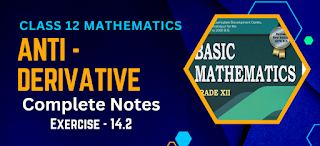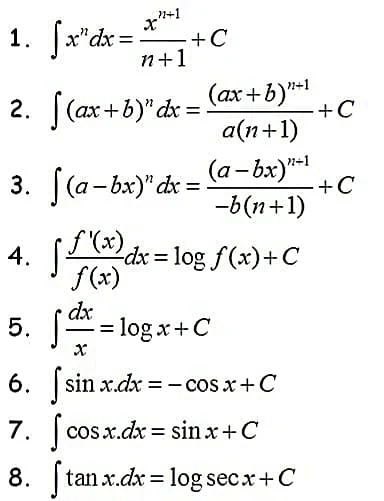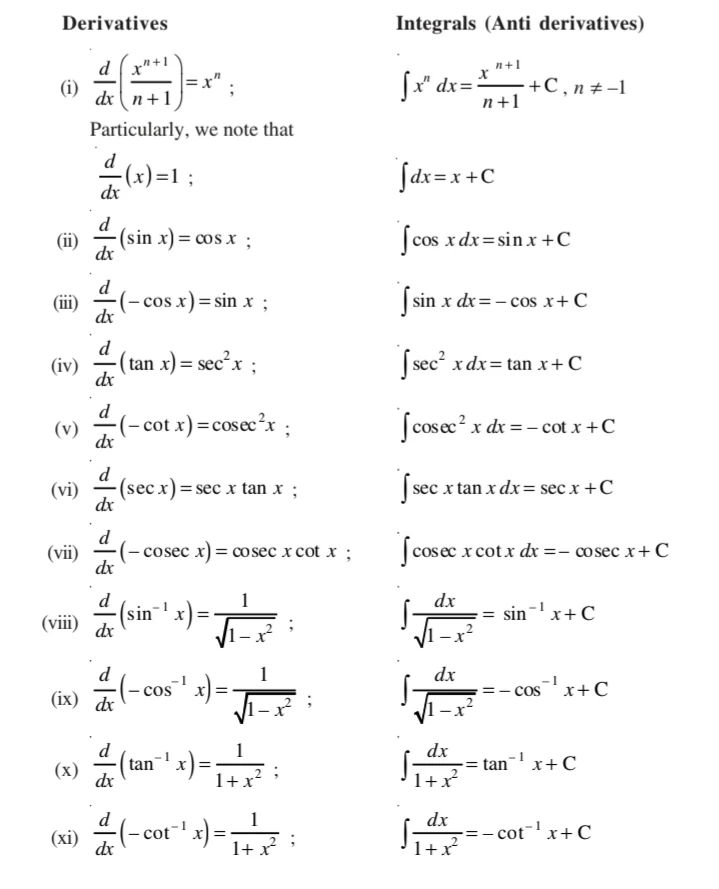# Basic Maths Class 12 Antiderivative Solutions | Exercise - 14.2Basic Maths Class 12 Antiderivative Solutions according to the latest syllabus of 2080. If you are desperately searching for class 12 antiderivative solutions PDF then in this article you'll get them. In addition to the solutions PDF, you'll also get the some important questions that may be asked for 1 marks during examination.

## Chapter - 14  Antiderivative

We already have a basic concept of antiderivative in class 11. We have solved many questions and got answers. Now in class 12 we use the answer that we got while solving antiderivative problems in class 11 as a formula to solve other questions in class 12.

We recently published the notes of first exercise of this chapter and students were demanding the notes of second chapter too. So we are here with the complete solutions of class 12 antiderivative second exercise. If you want the notes of first exercise then just click the link below.

### What is Antiderivative?

We all know that antiderivatives are the opposite of derivative. It is a function that reverses what the derivative does. One function has many antiderivatives, but they all take the form of a function plus an arbitrary constant. Antiderivatives are a key part of indefinite integrals.

In derivative we reduce the function to its lowers term while in antiderivative or integration we maximise the given lower terms. You'll get the clear idea about integration from the formulas given below.You'll need these formulas while solving the exercise. So, try to remember all the formulas we have studied in class 11 including the one given above.

Exercise - 14.2

This PDF will provide you each and every question solutions from the 1st exercise of class 12 antiderivatives chapter. If you want the solutions of other exercises then you can click on the respective exercises from above button.

Note: Scroll the PDF to view all solutions

### Standard Int.egrals (II)

In this section we consider the second set of standard integrals which can be evaluated by a technique known as integration by parts. This is a formula which can be deduced as given below.

We know,
d/dx (u×v₁) = du/dx (v₁)+ (u) dv₁/dx

### Comparison between differentiation and integration1. Both are operations on functions.
2. Both satisfy the property of linearity
3. We have already seen that all functions are not differentiable. Similarly, all functions are not integrable. We will learn more about nondifferentiable functions and nonintegrable functions in higher classes.
4. The derivative of a function, when it exists, is a unique function. The integral of a function is not so. However, they are unique upto an additive constant, i.e., any two integrals of a function differ by a constant.
5. When a polynomial function P is differentiated, the result is a polynomial whose degree is 1 less than the degree of P. When a polynomial function P is integrated, the result is a polynomial whose degree is 1 more than that of P.
6. We can speak of the derivative at a point. We never speak of the integral at a point.
7. The derivative of a function has a geometrical meaning, namely, the slope of the tangent to the corresponding curve at a point. Similarly, the indefinite integral of a function represents geometrically, a family of curves placed parallel to each other having parallel tangents at the points of intersection of the curves of the family with the lines orthogonal (perpendicular) to the axis representing the variable of integration.
8. The derivative is used for finding some physical quantities like the velocity of a moving particle, when the distance traversed at any time t is known. Similarly, the integral is used in calculating the distance traversed when the velocity at time t is known.

## Some Important Questions

As I mentioned earlier, I'll be providing you some of the important questions answers that might be asked in your class 12 final exams. We already have discussed about 3 important questions in our previous exercise but here we'll be doing some extra new questions.

If you want to view the important questions of previous exercise then you can visit the first exercise solutions from the link given above. After you go the that page, just scroll down ans you'll see important questions collection at the end of the article.

### 1. What is the standard formula for integration?

The standard formula for integration, also known as the power rule or the basic integration formula, is as follows:

∫x^n dx = (1/(n+1)) * x^(n+1) + C

In this formula, ∫ represents the integral symbol, x is the variable of integration, n is a constant exponent, dx represents the differential of x, C is the constant of integration, and ^(n+1) denotes raising x to the power of (n+1).

This formula is applicable when integrating a power function of x, where the exponent is not equal to -1. It provides a general method for finding the antiderivative or indefinite integral of such functions. Remember to add the constant of integration (C) at the end since indefinite integrals have an infinite number of solutions.

### 2. What is integration of 1?

The integration of the constant function 1 with respect to the variable x is given by:

∫1 dx = x + C

In this case, integrating the constant function 1 simply results in x, where x represents the variable of integration. The constant of integration, denoted by C, is added at the end since indefinite integrals have an infinite number of solutions.

### 3. What is the antiderivative commonly called?

The standard form in integration is generally considered to be the indefinite integral or antiderivative of a function. The antiderivative of a function represents a family of functions that differ by a constant value, known as the constant of integration.

The standard form is often represented as ∫f(x)dx = F(x) + C, where f(x) represents the integrand, F(x) represents the antiderivative, dx represents the differential of x, and C represents the constant of integration.

This form is useful for finding the indefinite integral of a wide range of functions, including polynomial functions, trigonometric functions, exponential functions, and more. By finding the antiderivative of a function, we can determine the original function that was differentiated to obtain the given function.

No Comment